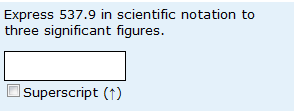# Significant figures and rounding

I’ve posted before about the difficulties students seem to have with significant figures and rounding. This post adds some data, to give you an appreciation of the size of the problem.The data are for the question shown on the left (from a summative iCMA, with all the implications that has as to students trying their best etc. – and the findings are very similar for all variants).  In order to answer the question the students have to write a number in scientific notation to three significant figures. In doing this, they have to round the number up. Of the responses analysed:

323 (90.2%) of responses were given in scientific notation

308 (86.0%) of responses were given to three significant figures

270 (75.4%) of responses had been rounded up.

The obvious conclusion here is that students are better at using scientific notation than at giving an appropriate number of sig figs – and rounding is a real problem. Some care is needed in interpretation,  since students who give an answer to 4 sig figs (as in the original value) didn’t have the opportunity to round up. So let’s look at individual errors:

7.2% of responses gave an answer in correct scientific notation but to 4 sig figs

7.2% of responses gave an answer in correct scientific notation but truncated to 3 sig figs rather than rounded up

4.7% of responses were numerically correct but not in scientific notation.

1.2% of responses were not in scientific notation and truncated to 3 sig figs rather than rounded up.

So it does indeed appear that significant figures and rounding present real difficulties for a significant minority of our students.

This entry was posted in mathematical misunderstandings and tagged , , . Bookmark the permalink.